# Multiple choice questions on EMF and Electric measurements(Electric Circuits)

## Multiple Choice Questions

Question 1
For accurate measurements, the resistance of a voltmeter should be
1. infinite
2. as small as possible
3. as large a possible
4. equal to the resistance across which potential difference is to be measured

Voltmeter when connected across any circuit element should not draw any current from it. If the voltmeter resistance is not high as compared to the resistance of the circuit element, across which it is connected, the measured voltage becomes less. Thus resistance of the voltmeter should be as large as possible.
Hence (c) is the correct answer

## Paragraph Type Questions

(A)Use figure given below for questions 2 to 4
Consider the circuit given below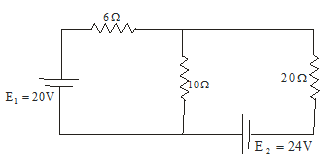Question 2
The rate at which energy is dissipated is 20Ω resistor
(a) 14.2 Watt
(b) 8.97 Watt
(c) 97.7 Watt
(d) 47.2 Watt
Question 3
Current through 10Ω resistor is
1. 1.54 A
2. 0.67 A
3. 1.22 A
4. 2.21 A
Question 4
Power of battery having emf E1=20V is
1. 44.2 Watt
2. 30.8 Watt
3. 13.4 Watt
4. 20 Watt

(i) Consider the circuit given in the question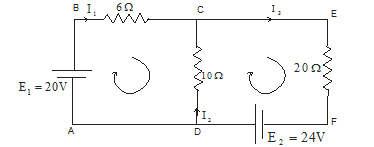Rate at which energy is dissipated in 20 Ω is
P=I2R
So we need to calculate the current through 20 Ω resistor. Applying Kirchoff’s loop rule in ABCDA we get equation
E1 -6I1 +10 I2 =0
or
20 -6I1 +10 I2 =0  ----(1)
Applying Kirchoff’s loop rule in CDEFC we get equation
E2 -10I2 -20I3  =0
24- 10I2 -20I3  =0        ---(2)
From Kirchoff’s junction rule at point C
I1 + I2 = I3
putting this in equation 2 we find
24-30I2 -20I1 =0       -----(3)
Solving equation 1 and 3 for the value of I1 we get
I1=2.21A
Again from equation 1 we find
I2=-0.67A here consider reversing the direction of current from the assumed one.
And finally
I3=1.54A
Rate at which energy is dissipated in 20 Ω is
P=I2R=(1.54)2x20=47.2 Watt
Hence (d) is the correct answer
(ii) I2 is the current through 10Ω resistor
Hence (b) is the correct answer
(iii) Power of battery is
Pemf=iE
Power supplied by battery E1 = I1E1=44.2 Watt

## Multiple Choice Questions

Question 5
Consider the figure given below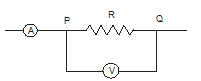If ammeter shows a reading of 10A and voltmeter having internal resistance 3000 Ω measures a voltage of 200V, the resistance R is given by
1. 201.3 Ω
2. 2013 Ω
3. 20.13 Ω
4. 20.00 Ω

Applying Ohm’s law in section PQ
V=IR
The resistances, R and internal resistance of the voltmeter are connected in parallel
$\frac {I}{V} =\frac {1}{R} + \frac {1}{R_v}$
or
$\frac {1}{R} = \frac {I}{V} - \frac {1}{R_v} = \frac {R_v I -V}{VR_v}$
Thus
$R=\frac {V}{I - \frac {V}{R_v}}$
putting the respective values and calculating we find R=20.13Ω

Question 6
A constant voltage is applied between the two ends of a uniform metallic wire. Some heat is developed in it. The heat developed is doubled if
(a) both length and radius of wire are halved
(b) both length and radius of wire are doubled
(c) the radius of wire id doubled
(d) length of wire is doubled

$H=\frac {V^2 t}{R}$
but
$R=\frac {\rho L}{A} =\frac {\rho L}{\pi r^2}$
Hence
$H=\frac {V^2 t r^2 \pi}{\rho L} Hence (b) the correct answer Question 7 If a wire of resistance 2Ω is covered with ice and a voltage of 210V is applied across the wire , then rate of melting ice would be 1. 0.85g/s 2. 1.92g/s 3. 6.56g/s 4. none of the above Answer Rate at which heat is produced in the wire is$\frac {heat}{time} =\frac {V^2}{R} = 2205 Js^-1$Latent heat of ice=80c/gm=80x4.2J/gm Hence rate of melting ice would be =$\frac {2205}{80X4.2} =6.56g/s$Hence (c) the correct answer Question 8 Which of the following statements is/are correct? 1. Both Peltier and Joule effects are reversible 2. Both Peltier and Joule effects are irreversible 3. Joule effect is reversible and Peltier effect is irreversible 4. Joule effect is irreversible and Peltier effect is reversible Answer (d) is the correct answer Question 9 The arrangement shown below is of the meter bridge experiment. Here AC=x corresponds to the null deflection in the galvanometer. What will be the value of AC if the radius of the wire AB is doubled?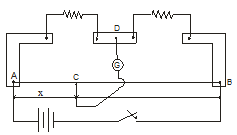1. x/2 2. x/4 3. 2x 4. x Answer When radius of the wire is changed , it will not affect the ratio of resistance of two arms AC and BC of the slide wire bridge. Hence (d) is the correct answer ## Assertion Reason type questions 1. Both assertion and reason are true and reason is correct explanation of assertion 2. Both assertion and reason are true and reason is not the correct explanation of assertion 3. Assertion is true but reason is false 4. Both assertion and reason are false. Question 10 Assertion The distribution of currents within the network for a given amount of current always takes place in a way that leads to minimum total power distribution in the circuit Reason For maximum output current by a source the source resistance must be equal to external load resistance. Question 11 Assertion When temperature of a cold junction of a thermocouple is lowered , the value of neutral temperature of this thermocouple rises Reason More thermo emf is produced when temperature difference between two junctions of thermocouple is raised. Question 12 Assertion A domestic appliance, working on a three pin , will continue working even if the top pin is removed Reason Third pin is used as a safety device. Question 13 Assertion A potentiometer of larger length is used for accurate measurements Reason Potential gradient for potentiometer of larger length with a given battery becomes small. Answer 10 (b) 11.(d) 12 (a) 13 (a) ## Multiple Choice Questions Question 14 12 wires each of resistance RΩ are connected in the form of a skeleton cube as shown below in the figure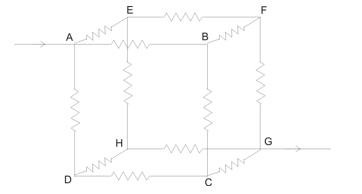If current I enter at point A and leaves at diagonally opposite point G then the equivalent resistance of the cube would be (a)12R/5 (b)12R (c) 6R/5 (d) 5R/6 Answer Given that current I enter the cube at point A and leaves at point G as shown below in the figure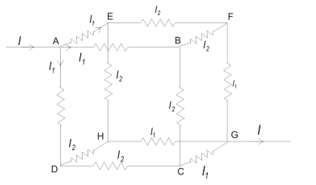Here we have I1=2I2 And also from point rule we have I=3I1 If E is the emf connected across the system then E=IReq Applying Kirchhoff’s law to any path between A and G , say ABFG , we get I1R+I2R+I1R=V=IReq Putting I1=2I2 in above equation we get 5I2R=IReq Since I=3I1 so we have I=6I2 From this we have 5I2R=6I2Req Or, Req=5R/6 ## Linked comprehension type question (B) Consider the figure given below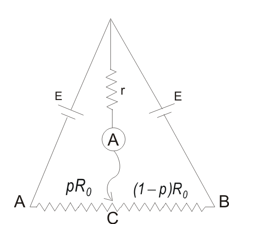Here AB is a wire of total resistance R0 . A jockey connected at point C can divide the wire into resistors of resistance pR0 and (p-1)R0 . Assuming that the batteries are identical and have zero internal resistance Question 15 Current through ideal ammeter for any 0<p<1 is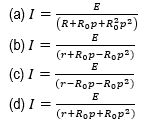Answer Let I be the amount of current through the ammeter, I1 be the current due to left hand EMF and$I_2$be the current due to right hand emf. Applying Kirchhoff's law we find$E-Ir-I_1pR_0=0E-Ir-I_2(1-p)R_0=0$and$I=I_1+I_2$Solving for I we get$I=\frac{E}{\left(r+R_0p-R_0p^2\right)} $Question 16 Value of p for which ammeter reads maximum value is (a) 0 (b) 1 (c) 2 (d) 3 Answer For maximum I denominator should be smallest which is for two values of p i.e., for p=0,1. (C) Consider the circuit given below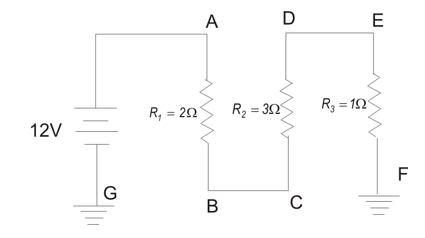Question 17 Total resistance in the circuit is (a) 5Ω (b) 6Ω (c) (11/6)Ω (d) 3Ω Answer From the figure in the question we see that all the resistors are connected in the series combination. So equivalent resistance of the circuit is$R=R_1+R_2+R_3 = 6$ω Question 18 Current in the circuit is (a) 4A (b) 12A (c) 6A (d) 2A Answer Total current in the circuit is I=V/R = 12/6 =2A Question 19 Potential difference between points A and E is (a) 12V (b) 6V (c) 10V (d) 5V Answer For finding potential difference between points A and E we first need to find the effective resistance between these two points.$R_{AE} = 2+3=5$ω$V_{AE} = IR_{AE} = 10$V Question 20 Potential at point E is (a) 10 V above the ground (b) 10 V below the ground (c) 2 V above the ground (d) 2 V below the ground Answer$V_E$is the voltage across the resistor$R_3$. So,$V_E=R_3I = +2$V above the ground Question 21 Power supplied by the battery is (a) 12 W (b) 10W (c)22W (d)24W Answer Power supplied is P=VI = 24W ## Matrix match type question Question 22 Consider the two circuits given below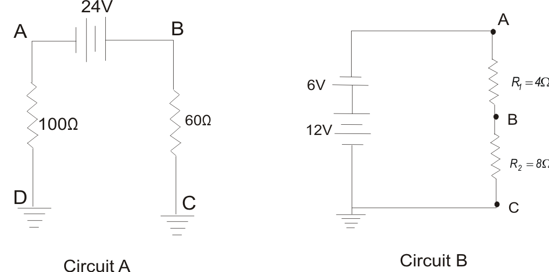Column A Column B (P) Point of lowest potential in circuit A has its value equal to (U) 0.15 A (Q) Circuit current in circuit B is (V) 4V (R) Circuit current in circuit A is (W) 15V (S) Potential of point B in circuit B is (X) 90V (T) Potential at point B in circuit A is (Y) .5A Answer First consider circuit A for finding value of lowest potential. Point of lower potential in circuit A is the point of maximum negative potential and its value is V=IR Where I= 24/160 = .15 A is the current in the circuit. So, Lowest potential is V=-0.15 × 100 = -15 V That is 15 V below the ground. Now potential at point B in circuit A = 0.15 × 60 =+9V Now we find circuit current in circuit B Net voltage= 12-6 = 6V Total resistance in the circuit = 4+8 = 12 ω And circuit current is, I =6/12 = .5A Now potential at point B in circuit B =$V_A-(4 \times 0.5)$Again potential at point A in circuit B =12-6=+6V which is positive w.r.t. ground$V_B = 6-2= +4V$P -> W ; Q -> Y ; R - >U ; S ->V ; T- >X ## Multiple Choice Questions Question 23 In the circuit shown below the reading of ammeter is the same when both switches open as both switches close.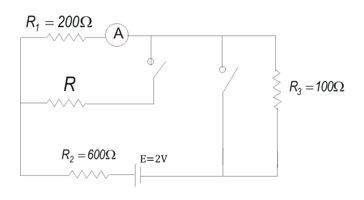The value of resistance R is (a) 800Ω (b) 900Ω (c) 1200Ω (d) 1000Ω Answer When both the switches are open resistances$R_1$,$R_2$and$R_3$are in series combination,So current through ammeter would be$I_A= \frac {E}{R_1 + R_2 + R_3}$Again with both Switches closed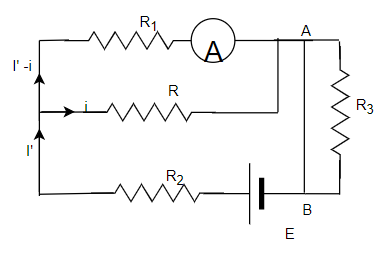Voltage at points a and b would be same and no current passes through resistance$R_3$. Now current through E and$R_2I'=\frac {E}{R_2 + \frac {RR_1}{R_1 + R}}$Current through Ammeter would be the current through resistor$R_1$. If i is current through resistor R then I' -i would be the current through$R_1$and ammeter. So from Kirchoff's law$(I' -i)R_1 = iR$or$i= \frac {I' R_1}{R+ R_1}$So current through ammeter$=I' -i = I' - \frac {I' R_1}{R+ R_1}= \frac {I'R}{R+R_1}$or$=\frac {RE}{(R+R_1)R_2 + RR_1}$Now since Ammeter reports same reading before and after$\frac {RE}{(R+R_1)R_2 + RR_1}=\frac {E}{R_1 + R_2 + R_3}R(R_1 + R_2 + R_3)=(R+R_1)R_2 + RR_1RR_1 + RR_2 + RR_3 = RR_2 + R_1 R_2 + RR_1$or$R= \frac {R_1}{R_2}{R_3} = \frac {200 \times 600}{100} = 1200$ω ## Linked comprehension type question (D) For questions from 24 to 26 consider the statement given below A 20µF capacitor which is initially uncharged is connected to a 6V battery through a resistor with resistance equal to 200Ω Question 24 Magnitude of final charge q0 on the capacitor is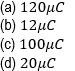Question 25 How long would it take for capacitor to be charged to (1/2)q0 after it is connected to the battery (a) 3.8×10-3s (b) 2.8×10-3s (c) 2.8×10-4s (d) 3.8×10-4s Question 26 The time taken by the capacitor to be charged to 0.80q0 is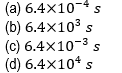Answer In charging of a capacitor we have$q=q_0(1-e^{-\frac{t}{RC}})$--- (1) Where$q_0 = CV$(a)$q_0=CV=\left(20\times{10}^{-6}F\right)\left(6V\right)=\ 120\mu C$(b) Time constant is given by$\tau=RC=\left(200\Omega\right)\left(20\times{10}^{-6}F\right)=4\times{10}^{-3}s$. Now from equation 1 if charge reaches${\frac{1}{2}q_0}$in time t then$ e^{-\frac{t}{RC}}=\frac{1}{2}$Taking log on both the sides and solving for t we get$t=t_{1/2}=2.8 \times 10^{-3} $s (c)$-\frac{t}{\tau}=ln 0.2$or,$\ t=\tau\times1.6=4\times{10}^{-3}\times1.6=6.4\times{10}^{-3}\ $s ## Matrix match type question Question 27 In the figure given below the circuit contains four capacitors having same capacitance of 10µF and a battery providing a voltage of 90V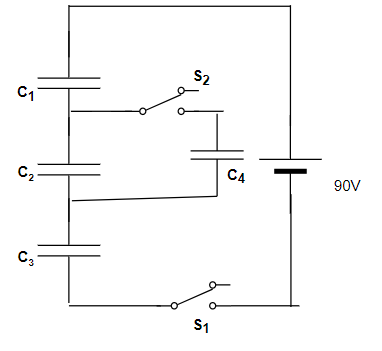Match the columns given below  Column A Column B (P) When switch S2 is open and S1 is closed and then opened after C1 , C2 and C3 are fully charged. Electric potential difference across each capacitor is (W) 0 V (Q) Now after opening S1 if switch S2 is closed, the electric potential difference across each capacitor is (X) V1=V3=36V , V2=V4=18V (R) In another case if switch S1 is open and switch S2 is first closed then electric potential difference across each capacitor is (Y) V=30 V (S) Now if switch S1 is closed , the potential difference across each capacitor is (z) V1=V3=30V , V2=V4=15V Answer First when switch$S_2$is open and$S_1$is closed the capacitor$C_4$is not included in the circuit and the effective circuit is shown below in the figure.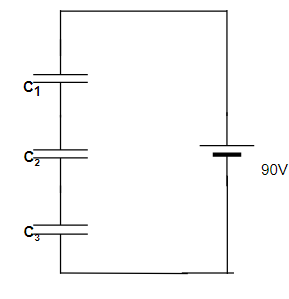If battery provides a voltage of 90V then$V_1+V_2+V_3=V$where$V_1$,$V_2$, and$V_3$are voltages across the capacitors$C_1$,$C_2$,$C_3$respectively. Since all the capacitors are in series combination so charge on all the capacitor plates is same say, Q. Also given that$C_1=C_2=C_3=C=10 \mu FQ=C_1V_1= C_2V_2= C_3V_3V_1=V_2=V_3=V'$which gives ,$3V'=V$or$V'=90/3 = 30$V Once the capacitors are charged this way, the potential difference across each capacitor will remain the same even after opening the switch$S_1$. If we now open switch$S_1$then battery is excluded from the circuit after charging of the capacitors$C_1$,$C_2$and$C_3$. Now closing switch$S_2$will bring$C_4$in the circuit and effective circuit would be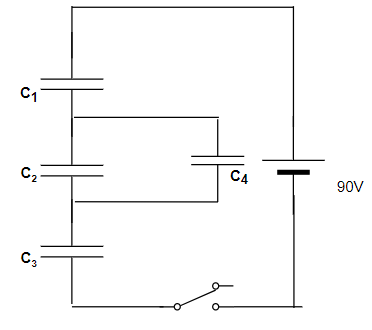Capacitors$C_1$and$C_3$will still carry the same charge as before and opening of switch$S_1$leaves them isolated from any flow of charge. Charge Q originally on capacitor$C_2$is distributed between capacitor$C_2$and$C_4$. As$C_2=C_4$so charge on each capacitor equals Q/2 and voltage across$C_2$and$C_4$would be equal to half the original voltage across C2. Thus$V_1=V_3=30V$,$V_2=V_4=15V$Again with capacitors initially uncharged and switch$S_1$open , closing$S_2$does not provide any potential difference across any capacitor as battery is effectively disconnected. Now we close$S_1$with$S_2$closed previously and circuit arrangement is as shown below in the figure.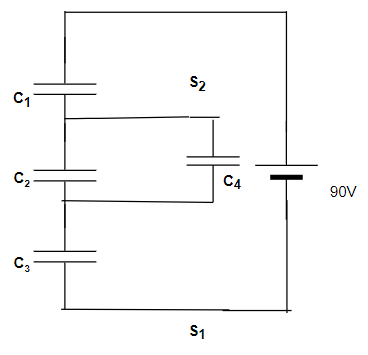Effective capacitance of parallel combination between capacitors$C_2$and$C_4$is$C_{24} = C+C = 2C$And the entire system has equivalent capacitance$\frac{1}{C_{eq}}=\left(\frac{1}{C_1}+\frac{1}{C_{24}}+\frac{1}{C_3}\right)$Or,$C_{eq}=\frac{2}{5}C$Thus, charge drawn from the battery onto each of$C_1$,$C_24$and$C_3$is,$Q'=C_{eq}V={\frac{2}{5}CV}V_1=V_3=\frac{Q'}{C}=\frac{2}{5}CV=36VV_2=V_4=\frac{Q'}{2C}=\frac{1}{5}CV=18V\$
Hence P ->Y ; Q -> Z ; R -> W ; S ->X

## Multiple Choice Questions

Question 28
Kirchhoff's junction rule is a reflection of
(a) conservation of current density vector.
(b) conservation of charge.
(c) the fact that the momentum with which a charged particle approaches a junction is unchanged (as a vector) as the charged particle leaves the junction.
(d) the fact that there is no accumulation of charges at a junction

(b), (d)